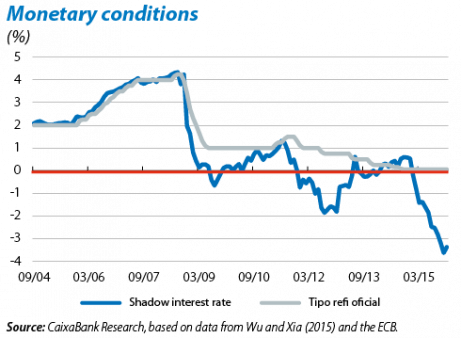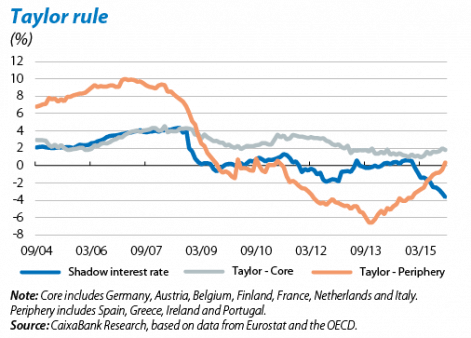# Discovering monetary policy in the shadow

Content available in
February 11th, 2016

Does the monetary policy stance meet the needs of the economy? Although it might seem trivial, in order to answer this question we need to know how to measure monetary policy. Before the Great Recession the interest rate at which central banks lend money to private banks (the Refi rate in the case of the ECB) provided a good description of how accommodative monetary policy was.1 However, in the last few years these interest rates have hit the zero lower bound and central banks in the advanced economies have started to use unconventional measures such as large-scale asset purchases. The central bank interest rate has therefore lost its informative power.

In the search for a new indicator of monetary policy, it has been suggested to use the historical relationship between the official Refi rate and a series of monetary variables to calculate the Refi rate we would observe in the present environment if this were not anchored at 0%: the so-called shadow interest rate. One of the most popular methodologies to calculate this is the one proposed by Wu and Xia (2015)2 who use a model of the term structure to project the shadow Refi rate. As we can see in the first graph, at times of standard monetary policy (up to the end of 2008) the shadow rate and the real Refi rate are practically indistinguishable. However, since the start of the financial crisis the shadow interest rate reveals some very useful information. Clearly the ECB's unconventional measures have eased monetary conditions beyond what is reflected in the official rate in 2009, 2012 and 2015. Moreover the shadow rate also points to a certain tightening of monetary policy with the withdrawal of these measures and the reduction in the ECB's balance sheet (in spite of lowering the Refi rate between December 2012 and the summer of 2014). Lastly, the announcement and implementation of QE by the ECB led to a dramatic easing of monetary policy in 2015, equivalent to a fall in the shadow interest rate from 0.54% in December 2014 to –3.38% in December 2015.

In addition to providing us with information on effective monetary conditions, the shadow interest rate also helps us to evaluate whether these conditions meet the needs of the economy using traditional tools such as the Taylor rule, which tells us what the benchmark interest rate should be according to the natural rate of interest, (core) inflation and the unemployment or output gap.3 The second graph shows how, before the financial crisis, monetary policy was much more in line with the needs of core countries than of those in the periphery. However, since 2009 the ECB's policy has found a middle point between both regions. During 2015, with the gradual recovery in core inflation and economic activity, the Taylor rule recommended less monetary relaxation, both in the core and in the periphery of the euro area. However, this rule is based on how the economy works under normal conditions and the current financial and economic situation is far from normal. Specifically there is uncertainty regarding the gap between the economy and its potential. This would suggest caution when interpreting the normalisation of monetary conditions recommended by this rule. On the other hand we must also take into account the fact that an environment with excessively low interest rates could lead to financial instability. All in all, it is reasonable to interpret the current discrepancy between the Taylor rule and monetary conditions as a sign that the latter should not be eased any further and the next step required by the economy is the gradual normalisation of the ECB's policy.

1. This has not always been the consensus opinion. For example in 1963 Friedman and Schwartz defended the use of monetary aggregates such as the M1.

2. Measuring the Macroeconomic Impact of Monetary Policy at the Zero Lower Bound, forthcoming in the Journal of Money, Credit and Banking.

3. Specifically$$\begin{array}{l}interest\;rate_t^{taylor}\;=\;r^{n\;}+\;\pi_t\;+\;\phi_\pi(\pi_t\;-\;\pi^\ast)\;+\;\phi_u(u_t\;-\;u_t^n)\\\end{array}$$, where $$r^n$$ = 2% is the natural rate of interest$$\pi_t$$ is current inflation and $$\pi^\ast$$= 2% the inflation target, $$u_t$$ is the unemployment rate and  $$u_t^n$$  the natural unemployment rate and  $$\phi_\pi$$ = 0.5 and $$\phi_u$$ = –1. See Nechio (2011), Monetary Policy When One Size Does Not Fit All, Federal Reserve Bank of San Francisco Economic Letter.